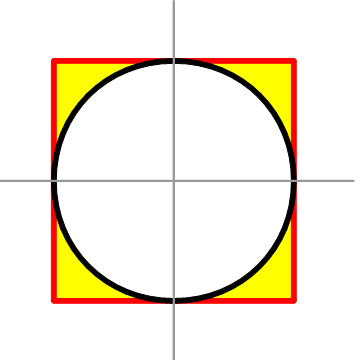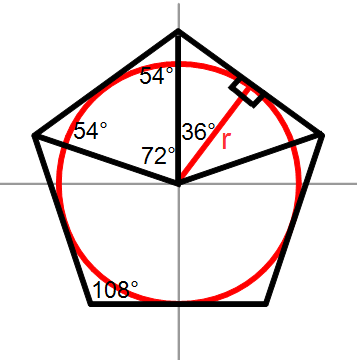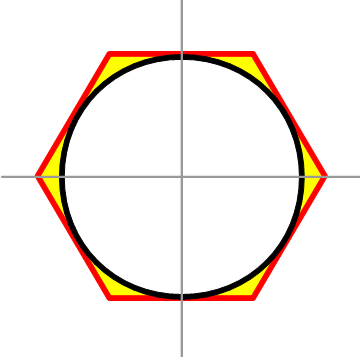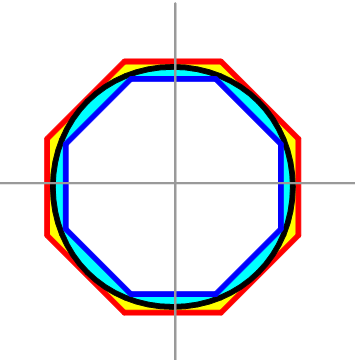# Thinking About the Value of Pi

The number pi (π) is far more than just an esoteric button on calculator keypads, little used by everyday people on the street, and thought useful only to scientists accustomed to the Greek alphabet. March 14 (3/14) is Pi day, and a good day to remember a little of that grade school mathematics that plays such an important role in so much of the world around us. Have you ever wondered why does π have the value it does, 3.14159265…? Not a little bit more, or a little bit less?  In this Pi day adventure you will see for yourself with a little arithmetic, algebra and Euclidean geometry (of the sort learned in high school) just how π comes out to be the number it is.

### A Simple Geometric Definition of π

The number we represent by the Greek small letter, pi (π), is defined as the ratio of a circle’s perimeter (more formally called its circumference) to its diameter (a line segment cutting the circle into two equally-sized half-circles, and in order to make the two half-circles exactly equal such a line must pass through the exact center of the circle). This definition will be adequate for all of our ensuing adventure.

### Calculating Perimeters

We are taught very early in school about the perimeter of polygons such as triangles, squares, and other n-sided polygons, usually in the context of word problems about fences, walls, boundaries, etc. If a square fence around our yard has four sides of equal length, s, then its perimeter is 4s. That is, if each side of the square fence is 15 meters long, then the perimeter of the entire square fence around the yard is:

4 sides X 15 meters/side = 60 meters

Circles are different in a special way from n-sided polygons. How are you supposed to calculate its perimeter (circumference), if for example, you need a circular fence around your flower garden? If you have a circle already, perhaps you could obtain its circumference by using a tape measure, or a string wrapped around the circle, that you can stretch out straight for measurement against a ruler. But suppose you don’t have such a circle already, and need to know how much fencing to purchase to encircle your garden?

This is where π comes in. You can measure the straight diameter of your garden, suppose it is 2.00 meters across. By multiplying this diameter by π you can calculate how much fencing you will need to buy.

2.00 meters X π = 2.00 meters X 3.14 = 6.28 meters

### Calculating Areas, Volumes and More …

Before continuing, it also should be mentioned that π doesn’t only have value in calculating the circumference of a circle. It comes up repeatedly throughout mathematics, science, engineering and in other problem domains. It also applies to the area contained within a circle, which is π X  where r stands for the radius (one-half of the length of the circle’s diameter, that is a line segment starting at the center of the circle and ending at the edge of the circle). So in the previously cited example of the circular flower garden with a diameter of 2.00 meters (a radius of 1.00 meter), we know the area within the garden is π square meters. This will be important when you need to figure out how much top soil, vermiculite and fertilizer to buy for your garden.

π X (1.00 meter)² = 3.14 X (1.00 meter)²  = 3.14 m²

You can also use π to calculate the volume of shapes which are in some way circular, such as cylinders and spheres. Take a can of chicken noodle soup as an example. When you buy a can of chicken noodle soup at your local grocery store, it may say it contains 628 milliliters of chicken noodle soup. How do you compute by measuring the can whether it actually contains the volume of chicken noodle soup it says on the label? Some of the volume within the can might be filled with an air pocket, but certainly if the can’s cylindrical volume is less than 628 cubic centimeters (cm³), it cannot contain 628 mL of chicken noodle soup, right? The formula for the volume of a cylinder is π X r² X h where h is the height of the cylinder. Here is one size of a cylinder which could contain 628 mL of chicken noodle soup,

π X (5 cm)² X  8 cm = 628 cm³
A cylinder can 10 cm across and 8 cm in height.

The applications of π in the circular shapes around us in our everyday lives, not to mention underpinning the science behind our technological gadgets, go on and on. But why is π the value that it is, 3.14159265 …? Is this value somehow a “magic” number?

### Approximating Pi with N-Sided Polygons

Long ago, geomancers believed shapes possessed “magical” qualities. They would inscribe certain shapes within other shapes, ascribing an almost mystical significance to them. We need not believe in anything so supernatural to use a little Euclidean geometry in finding the value of π. The technique I will use is based on the regular polygons we’ve already learned well in school, circumscribed around a unit circle.

A unit circle is just a circle on a Cartesian graph with its radius equal to 1 unit of length, and its center at the origin, (0, 0). If you have a sheet of graph paper, you might sketch such a circle 1 unit away from the origin, intercepting the coordinate axes at (1, 0), (0, -1), (-1, 0) and (0, 1).

All of the polygons I shall use are regular polygons with sides of equal length, and equal interior angles. When they circumscribe a circle, they appear on the outside of the circle but touch it at exactly one point for each side. Since these points are touching the unit circle, they are by definition exactly 1 unit of length from the origin. Since each polygon is regular, the points at which its sides touch the inscribed circle are at the midpoint of each side.

The first circumscribed shape I will consider is a square, with its four sides of equal length 2. If you’re uncertain why each side of this square must be length 2, consider that for it to circumscribe the circle its vertical lines must intersect with the circle at (-1, 0) and (1, 0), which are a distance 2 units of length apart. Similarly, its horizontal lines must intersect the circle at (0, 1) and (0, -1) which are also a distance 2 units of length apart. Therefore, each side of the square circumscribing the unit circle must be of length 2.Fig 2. The unit circle circumscribed by a square with the difference in area highlighted.

Figure 2 shows the difference between the area of the square and that of the unit circle shaded in yellow. The square’s area is s² = (2)² = 4.  Meanwhile, the area of the unit circle is π. We can see that 4 is close to 3.14… if we’re playing horseshoes. What about the perimeter? The square’s perimeter is 4s = 4(2) = 8.  The circle’s circumference is 2π = 6.28… Close again, but can I quantify how close I am?  Observe that you can find the area of the square is approximately 0.86 greater than the unit circle by s² – πr² (that is, subtracting the area of the circle from that of the square to get the area of the yellow shaded area). If I’m getting closer, this “error” in using a polygon to approximate the value of π should diminish.

My next circumscribed shape is the regular pentagon. I figured that if 4 sides is close, 5 sides should approach π even more closely. First, I sketch these two shapes and color-in the difference in area between the circumscribed regular pentagon and unit circle. Visually, it looks like the area that I’ve shaded appears to be less than it was with the square, doesn’t it?

In order to be certain that I’m going in the right direction, I want to follow the same procedure as I did with the square. This will require computing the area and perimeter of the regular pentagon, and that’s going to be more involved than in the case of the square. To obtain these numbers for the pentagon, I’m going to have to carve it up into right triangles as illustrated by Figure 4.  It turns out that my regular pentagon can be formed from ten of these right triangles.Fig 4. A regular pentagon can be divided into 10 right triangles with a height r and an interior angle 36°.

Right triangles permit me to use the trigonometric functions to calculate the length of the leg opposite the 36° interior angle. This length (tan 36°) is one-half the length of a side of my regular pentagon. Since r = 1, s = 2(tan 36°) = 2(0.727) = 1.45, and the perimeter of my regular pentagon, P = 5s = 5(1.45) = 7.27. This puts me closer to the circumference of the circle (6.28) than the perimeter of my square (8) had.

I can similarly calculate the area of each of these right triangles to obtain the area of my regular pentagon. Each triangle has an area of ½bh = 0.3635where b is the base (0.727), and h is the height (r = 1) of the triangle. Since there are ten of these triangles, the total area within my regular pentagon is 3.64, which compares more closely to the area of the unit circle (3.14) than my square (4) did. I’ve therefore calculated the yellow shaded area in Figure 3 to be approximately 0.50.Fig 5. A regular hexagon circumscribing a unit circle approaches its area even more closely.

The next circumscribed polygon I will show you is a regular hexagon with 6 sides. I will not step-through these computations, but observe that a similar triangular approach to that which I had used in the case of a pentagon remains applicable. In this case, the regular hexagon nicely forms a set of twelve 30-60-90 triangles, so that you can use the 30-60-90 triangle rule in which the sides are in the ratio 1 : √3 : 2.

The perimeter of this hexagon is 4√3 =  6.93, which is closer to that of the circle (6.28) than the pentagon (7.27) had been. The area of this hexagon is 2√3 = 3.46, which is closer to that of the circle (3.14) than the pentagon (3.64) had been. The difference in area shaded in yellow is now down to roughly 0.32.

So it is that as you draw regular polygons with ever-increasing numbers of sides, n, which circumscribes the unit circle, you will approach the numerical value of π from above. Always getting a closer approximation, as close as you care to make it, but never quite reaching π. In Figure 6 you can see the regular 8-sided octagon approach even nearer, but whether I take n = 8 sides, or n = 1,000,000 sides, I will not quite reach the value of π (although in the latter case, a number of the first digits will match).Fig 6. A regular octagon circumscribed around the unit circle with difference in area shaded yellow. Another octagon inscribed within the unit circle with its difference in area shaded in light blue. The value of π can be approximated from above and below.

Up until now, I have circumscribed a regular n-sided polygon on the outside of the unit circle, obtaining values that approach π from above, but that are always slightly greater than π. Figure 6 demonstrates that one can also approximate the value of π using an inscribed regular n-sided polygon. If I were to perform these computations, they would similarly approach π more and more closely, but from below. In each case, the approximate area and perimeter would be less than π.

### Taking the Limit

If this approximation process makes you think of left-sided and right-sided limits in calculus, then you’re right. As n→∞, so does the area of the n-sided regular polygon approach the value π from the right (if inscribed) or the left (if circumscribed). However, one does not need to have an appreciation of calculus to appreciate the number π. In fact, if you’ve never heard of taking a limit until now, then this illustration of approximating what the number π is should be an enlightening preview.

### Conclusion

Pi Day is a great day on which to remember and celebrate the mathematics and problem-solving techniques we learned in school. The applications of the number π have ramifications on our everyday lives, but many of us never pause to reflect on its significance. It doesn’t have to be a cryptic symbol placed out-of-reach, but instead can be rediscovered by anyone.